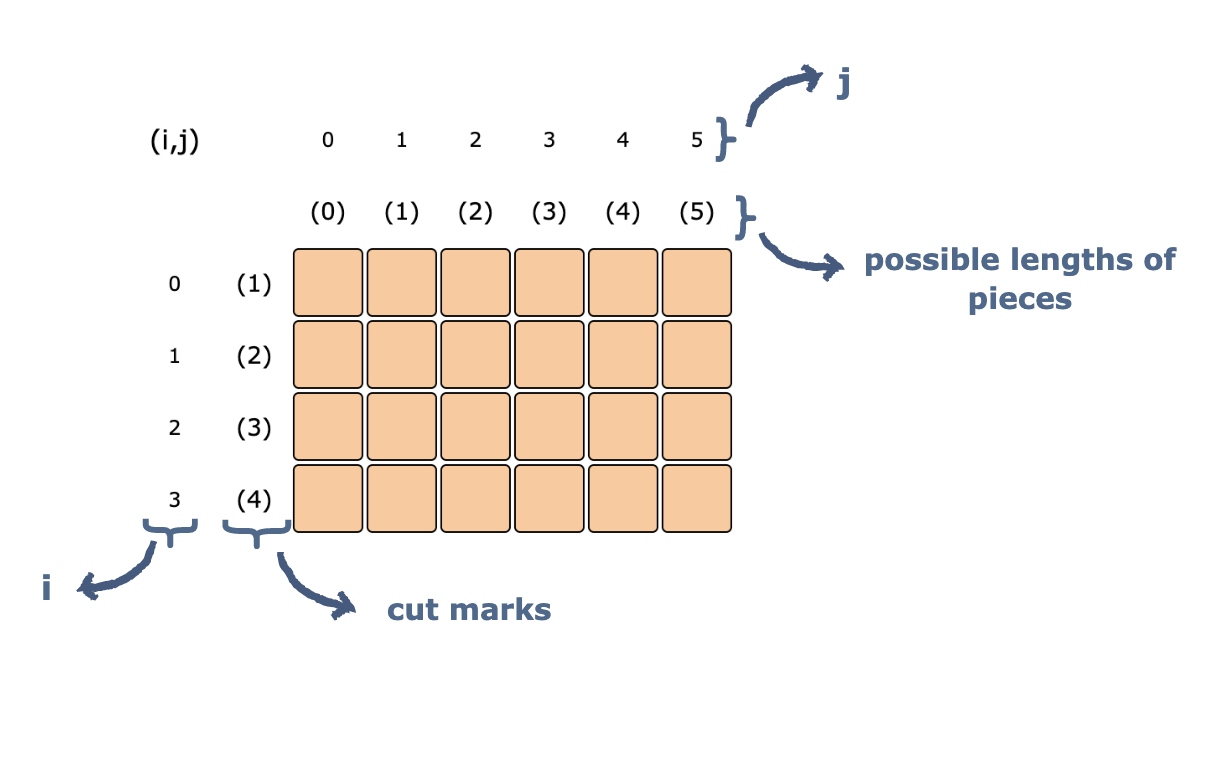Related Tags

python
java
c++

# The rod cutting problemEducative Answers Team

## Problem statement

Given a rod of length n, and an array that contains the prices of all the pieces smaller than n, determine the maximum profit you could obtain from cutting up the rod and selling its pieces.

Suppose that we have a rod of length 5, and an array containing the length(1,2,3 and 4 ) and price(2,5,7 and 8 ) of the pieces.There are various ways to cut the rod into sub-rods, each way results in a certain profit.The answer should be 12 (selling the sub-rods of length 1+2+2 gives a 2+5+5 profit).

A naive solution for this problem would be to generate all the configurations, of all the different pieces, to find the highest priced configuration. However, this method is exponential in time complexity.

We need to come up with a much​ better algorithm.

## Using dynamic programming

Since dynamic programming is mainly an optimization-over-plain recursion, we can use it to optimize the above exponential time algorithm. The idea is to store the results of subproblems so that we do not have to re-compute them when they are needed.

So, in the above example:

• The possible range of length, of any sub-rod, is from 0 to 5. A sub-rod of a length within this range​ can be made.
• We have 4 different prices of different-length sub-rods (i.e.,​ cut marks, the place where cuts can be made on a sub-rod).The grid above represents our sub-problems. Let:

• i be the number of rows
• j be the number of columns

The cell at [i,j] represents the maximum amount of profit that can be earned from selling a rod of initial length j. One is able to choose the cuts from an array of 0 to i.

For example, the cell at [2,3] represents the maximum profit that can be earned from a rod with length 3, and an array of different prices of different-length sub-rods(0 to 2).

Now, let’s start filling in the table/array.

Start with the simple calculations first. If a rod has a length of 0, what would the maximum profit be? It would be 0​.### Now, let’s start to fill in the rows

• In the first row, we only have the price 2 at a length of 1 in our array.
• At index [0,1], putting a cut at 1 would give a profit of 2. No cut would give a profit of 2.
• At index of [0,2], putting a cut at 1 would give a profit of 4 (2 rods with a length of 1, each with a price of 2). No cut would give a profit of 4 (not 5 because the price of 2 does not exist in the array yet!). Hence, 4 is the better option.
• Do this for the whole first row. ​

Your grid should look like this now:You may have observed that we’re deciding, at every step, whether the newly added cut mark ( i+1) increases the profit of the given rod j, or not. We decide this by taking the maximum of 2 things:

• The maximum profit of the length before the cut mark was made (i.e., the value at [ i-1,j] ). This is a case where we don’t include the newly added cut mark.
• price [i] + the maximum profit at length j-i-1 for the current array of prices (i.e., the value at [i, j-i-1]) would have already been computed before this computation. This is a case where we use the new cut mark for its effect on the profit.

So, the general expression used for filling the grid is:T is the grid, and price is the array of prices.

In the cases where j <= i, j-i-1 would be negative, simply use the value at [i-1,j]

Take a look at the example below; it shows how to use the expression to fill values in a grid:Once the grid is filled, which cell contains our answer? Let’s recall that the problem asked us to maximize the profit of a rod, of length n, with an array of prices from 0 to n-1. This is the cell in the extreme bottom-right:Since we already have an expression for filling in the grid, putting this into code shouldn’t be too difficult.

See the code below:

# Using numpy arrays
import numpy as np

price = [2,5,7,8]
n = 5

# Make an initial grid of all zeros
T = np.zeros((len(price),n+1))

for i in range(0,len(price)):
for j in range(0,n+1):

# First column => 0 length of rod => 0 profit
if j == 0:
continue

# First row => T[i-1,j] doesn't exist so just pick the second value
elif i == 0:
T[i,j] = price[i] + T[i, j-i-1]

# where j <= i => T[i, j-i-1] doesn't exist so just pick the first value
elif (j-i-1) < 0:
T[i,j] = T[i-1,j]

# using the whole expression
else:
T[i,j] = max(T[i-1,j], (price[i] + T[i, j-i-1]))

# Answer in the extreme bottom right cell
print "Maximum profit is", T[len(price)-1,n]



This algorithm has a time complexity of $O(n^2)$, which is polynomial.

RELATED TAGS

python
java
c++